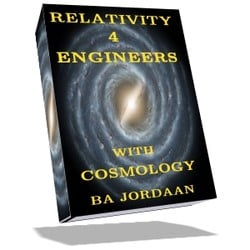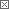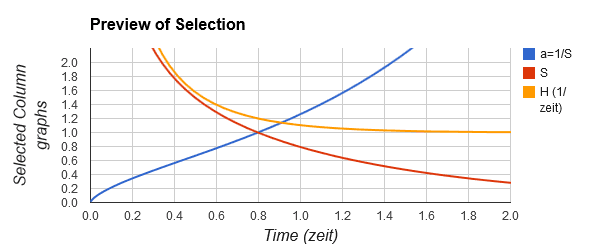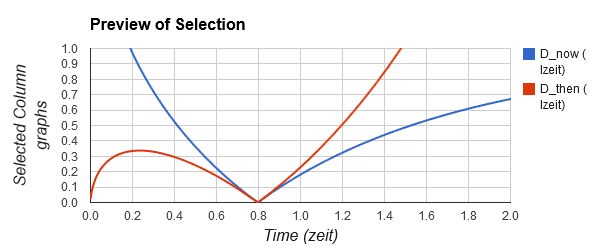Relativity and Cosmology Blog# Relativity and Cosmology

This is a Blog on relativity and cosmology for engineers and the like. My website "Relativity-4-Engineers" has more in-depth stuff.

Comments/questions of a general nature should preferably be posted to the FAQ section of this Blog (http://cr4.globalspec.com/blogentry/316/Relativity-Cosmology-FAQ).

A complete index to the Relativity and Cosmology Blog can be viewed here: http://cr4.globalspec.com/blog/browse/22/Relativity-and-Cosmology"

Regards, Jorrie

 Previous in Blog: How Aeons turned into Zeons Next in Blog: Time Dilation and Lorentz Contraction Revisited# How Zeons turned into something Useful

Posted June 30, 2015 6:00 PM by Jorrie

In the last Blog, How Aeons turned into Zeons, we derived a very simple formula for the expansion dynamics of the cosmos, normalized to a 'natural cosmological timescale' unit of 1 zeit = 17.3 billion years.

(2.1) H2 = 1 + 0.443 S3

where H is the fractional expansion rate and S is the 'stretch-factor', i.e. by how much distances have 'stretched' since light we observe has been emitted. It is basically the redshift factor z plus 1. By convention, S have a present value of 1.0; hence the present Hubble value H0 = (1.44)0.5 = 1.2 zeit-1. But what is the relationship between H and cosmological time?

## Cosmological Time

Because of the instantaneous "interest on interest" nature of cosmic expansion, it follows an exponential pattern (eHt), provided that H remains constant over time. Presently H is still decreasing, so cosmological time is approximated by the following natural logarithmic equation:

(2.2) t = ln[(H+1)/(H-1)]/3 zeit.

Let us put our present H0 = 1.2 zeit-1 in here (using Google or some other calculator) and check that we get the 0.8 zeit that we expect.

t_{now} = ln[2.2/0.2]/3 ~ 0.8 zeit.

Suppose we observe the redshift from a specific galaxy as z=1, which means that the light has left it when the wavelength stretch factor was S = z+1 = 2. Plugging S = 2 into eq. 2.1, we find that H = 2.13 zeit-1 when the light was emitted. The cosmic time was

tthen = \ln[(2.13+1)/(2.13-1)]/3 = 0.34 zeit after the start of the present expansion.

The light from that galaxy obviously took 0.8 - 0.34 = 0.46 zeit to reach us - it is the so-called 'lookback time'.

Now that we have a feeling for the relationships, what is better than viewing it on a graph? Here you see the parameters mentioned so far (against time).You can see our present time (0.8 zeit) clearly where the red and blue curves cross, i.e. where both S and a are unity. You can also see how H starts high and asymptotically approaches unity. The graphs were plotted using LightCone 7zeit, a variant of the standard LightCone 7 cosmological calculator.

I have 'sneaked in' the curve for the scale factor (a=1/S), but I did not give a direct relationship of it against time yet. You get it by solving eqs. 2.1 and 2.2 simultaneously, which is not a simple exercise at all. Fortunately, mathematics comes to the rescue and we have a nifty solution for a(t), i.e. the scale factor as a function of time:

(2.3) a(t) = sinh2/3(1.5t)/1.3

where sinh is the hyperbolic sine function. The 'hypersine' is obviously related to the natural logarithm ln(x) and the natural exponent (ex). The factor 1.3 is just scaling to make anow = 1 at tnow = 0.8 zeit, as is the convention. The importance of a(t) is that its curve shows how a decelerating expansion that gradually changed over to an accelerating expansion. The inflection point can be visually judged to lie between 0.4 and 0.5 zeit. It is actually at 0.44 zeit.

## Proper Distance

Proper distance in cosmology is like measuring distance on a hypothetical frozen view of the cosmos, i.e. with expansion stopped. Obviously we cannot stop expansion, so how do we find proper distance? There are various methods, but once we have the present and long term Hubble values (H0 and H), we only need to measure the redshift of a source and then calculate its proper distance from where we are.

Because of the non-linear expansion, there is no precise analytical solution for the proper distance (that I know of). We have to numerically integrate in small steps; but fortunately the definite integral is rather neat:

(2.4) Dnow = ∫1S(dS/H) = 1S[dS/√(0.44S3+1)]

We have substituted H from eq. 2.1 in here, so that we have a distance in terms of the observable S = z+1. For calculation, you can use your favorite integrator, but a rather cool web based one is available at

For our previous sample galaxy at S=2, I entered 1/sqrt(0.443x^3+1) into the Function box, with 1 in the "From" and 2 in the "To" boxes and then clicked "Compute" - it returned the answer as 0.64, which in our cas has the units lightzeit. So we receive the light when the proper distance to the galaxy is Dnow = 0.64 lzeit. Since we have used stretch S = 2, the proper distance when the photons left the galaxy was half of 0.64, i.e. 0.32 lzeit; so we say that Dthen = 0.32 lzeit for this galaxy. Recall that the light left that galaxy at t = 0.34 zeit, i.e. 0.46 zeit ago.

Here is a graph of Dnow and Dthen.The red Dthen curve is also known as the cosmological lightcone. To the left of 0.8 zeit it represents our past lightcone and to the right of 0.8 zeit our future lightcone. Near t = 0.0 the universe was very dense and objects we now observe were actually very, very close to our space locality. Distances then grew much faster than the progress that photons could make in our direction and ancient photons were at first dragged away from us. At around t = 0.23 zeit, the fractional expansion rate dropped enough to allow photons to make progress and eventually reach our telescopes.

To summarize, we have now looked at a further three rather easy equations that allow us to calculate the most common values for the standard cosmological model.

Part 3 will deal with the Hubble radius and two cosmological horizons that we should take notice of.

=0=

End-notes

 The function eHt gives the factor by which cosmic distances increase in a time interval t, provided that H remains constant over this time interval. In the far future, H will be a constant, but in the early universe H changed significantly over periods longer than a million years. Hence the more complex general solution.

 To verify that the approximations are valid, I used the LightCone 7zeit calculator for a 'one-shot' calculation with Supper=2 and Sstep=0.

 The Dthen lightcone is where the LightCone 7 calculator got its name from.

Interested in this topic? By joining CR4 you can "subscribe" to